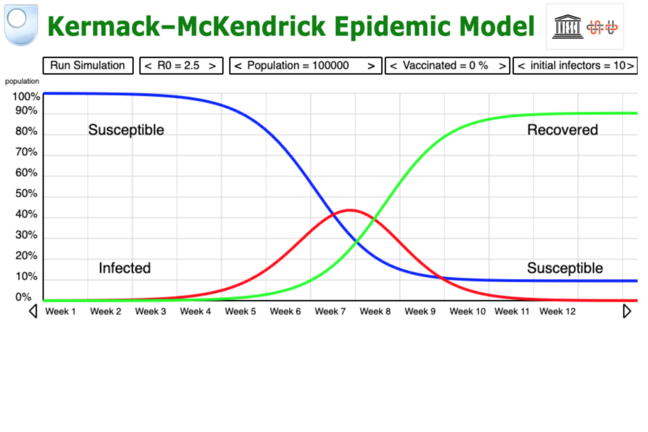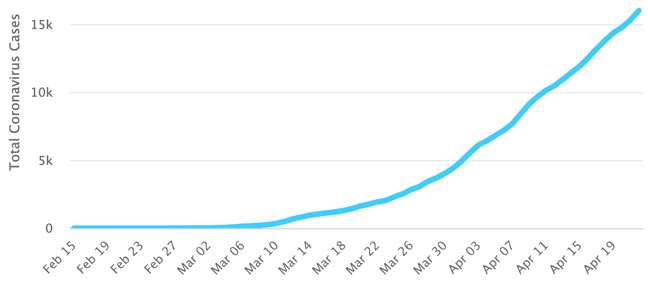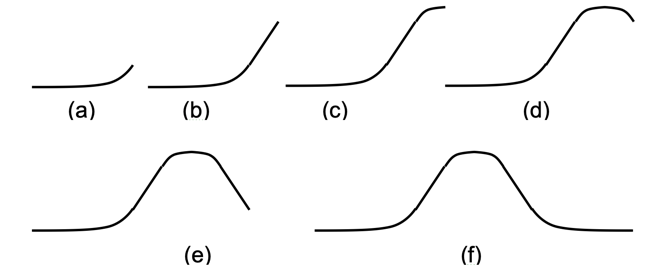## Want to keep learning?

This content is taken from the UNESCO UNITWIN Complex Systems Digital Campus & The Open University's online course, COVID-19: Pandemics, Modelling, and Policy. Join the course to learn more.
1.6

## UNESCO UNITWIN Complex Systems Digital Campus# The Shape of Epidemics

The first thing to do when modelling a real world system is to look at it and abstract the most important features. For epidemics the fundamental things is the population. Let this be represented by the symbol $$P$$.

For the COVID-19 system another fundamental feature is the people who are sick or die from the virus. At any point in time let the symbol $$I$$ represent the number of infected people.

The number of people infected can be found on the www.worldometers.info website. This site presents the World Health Organisation’s data for many countries in the world. The data for Sweden are shown in Figure 6.1.

Figure 6.1. Number of COVID-19 cases in Sweden to 22nd April 2020.

Assume that “When a person gets infected by a virus, they create antibodies that kill the virus and this enable them to recover. These antibodies prevent further infection”. In other words the people who have recovered from a virus cannot be reinfected. According to fullfact.org, an independent fact-checking website, it’s very rare to catch Covid-19 twice. This means there is a set of people who have recovered from the virus. Let this be represented by the letter $$R$$.

What about the people who are not infected and not recovered? They are susceptible to being infected. Let the set of these people be represented by the letter $$S$$.

Already we have enough to formulate a simple model:

Let $$P$$ be the population at the start of an epidemic

Let $$I$$ be the number of people infected on a given day

Let $$R$$ be the number of people recovered on that day

Let $$S$$ be the number of people who are susceptible on that day.

Then $$P = S + I + R$$.

Already this “SIR” model can tell us something about epidemics. Initially assume that $$I$$ begins to increase rapidly in time (Figure 6.2(a)). A UK Government leaflet suggests that after nine days people have recovered and are no longer infectious .Since $$R$$ increases and $$I$$ decreases, $$I$$ can’t keep rising so steeply and has to flatten off (Figure 6.2(b)) and then turn down until it’s horizontal (Figure 6.2(c)). Then by a similar argument it has to turn down until the number of infected people is around zero Figure 6.2(d)(e)(f)).

Figure 6.2. Building up the infected curve for epidemicsBy making the assumption that day by day the numbers change smoothly, this curve is be similar to the curve shown at the top of the page. So, by making a few assumptions we have built a simple mathematical model, $$P = S + I + R$$, that is close to the classic Kermack–McKendrick model of epidemiology. This is the curve that everyone talks about flattening.

## What do you think

If you never built a model before do you think you could follow this simple approach? Do you think that this model may be too simple to be useful?

## Reference

 fullfact.org, It’s very rare to catch Covid-19 twice, viewed 23rd April 2020. https://fullfact.org/health/coronavirus-catch-twice/